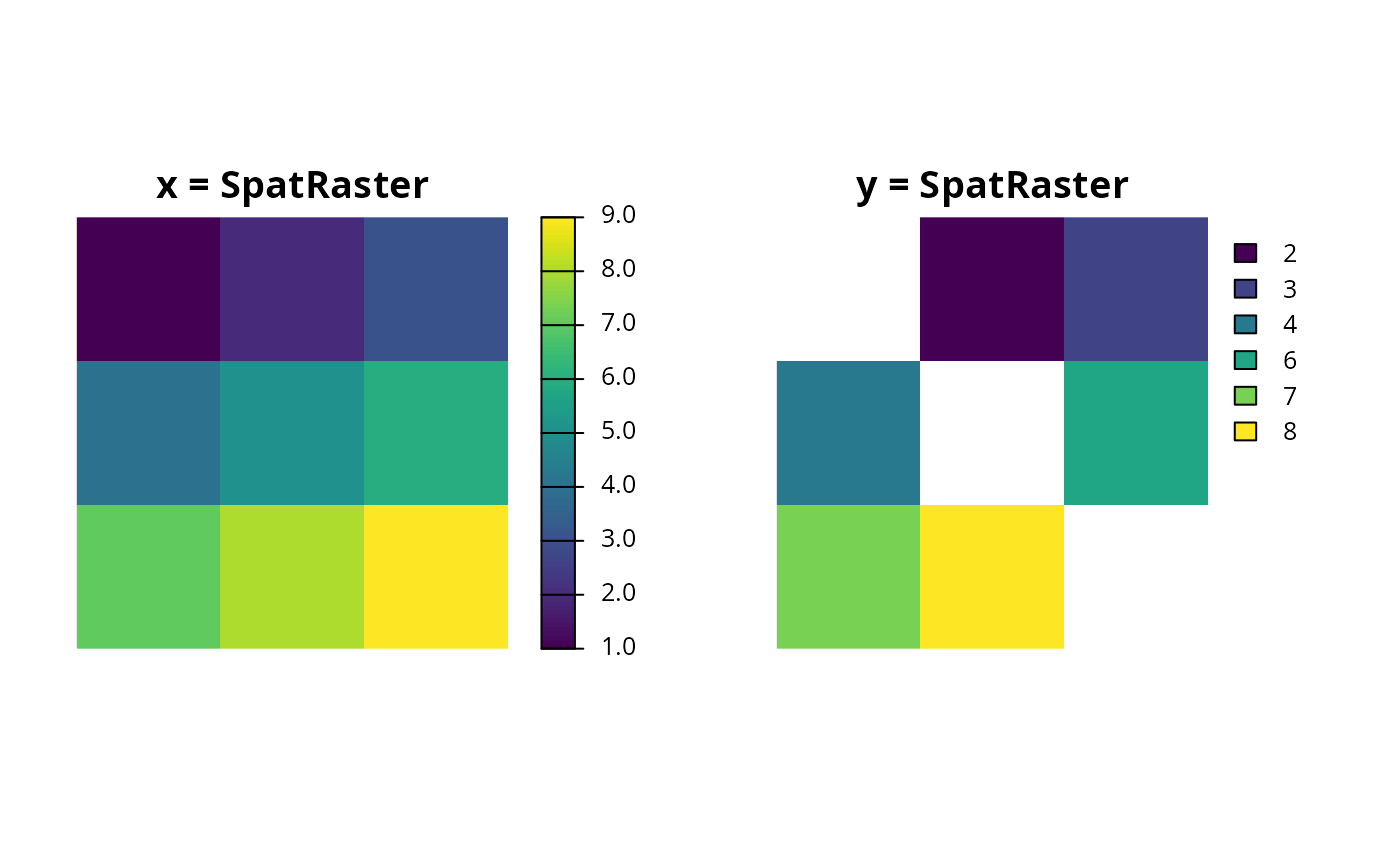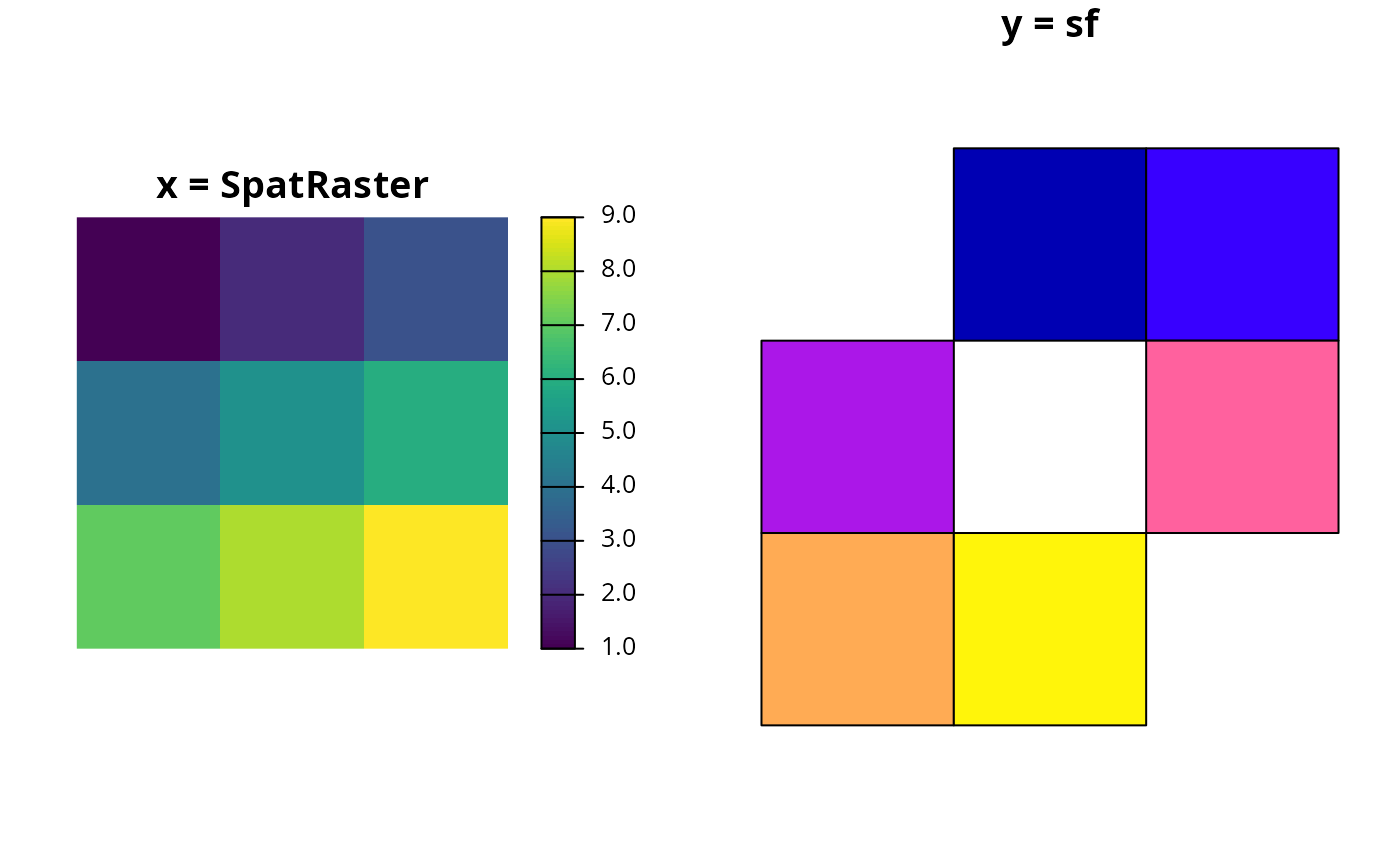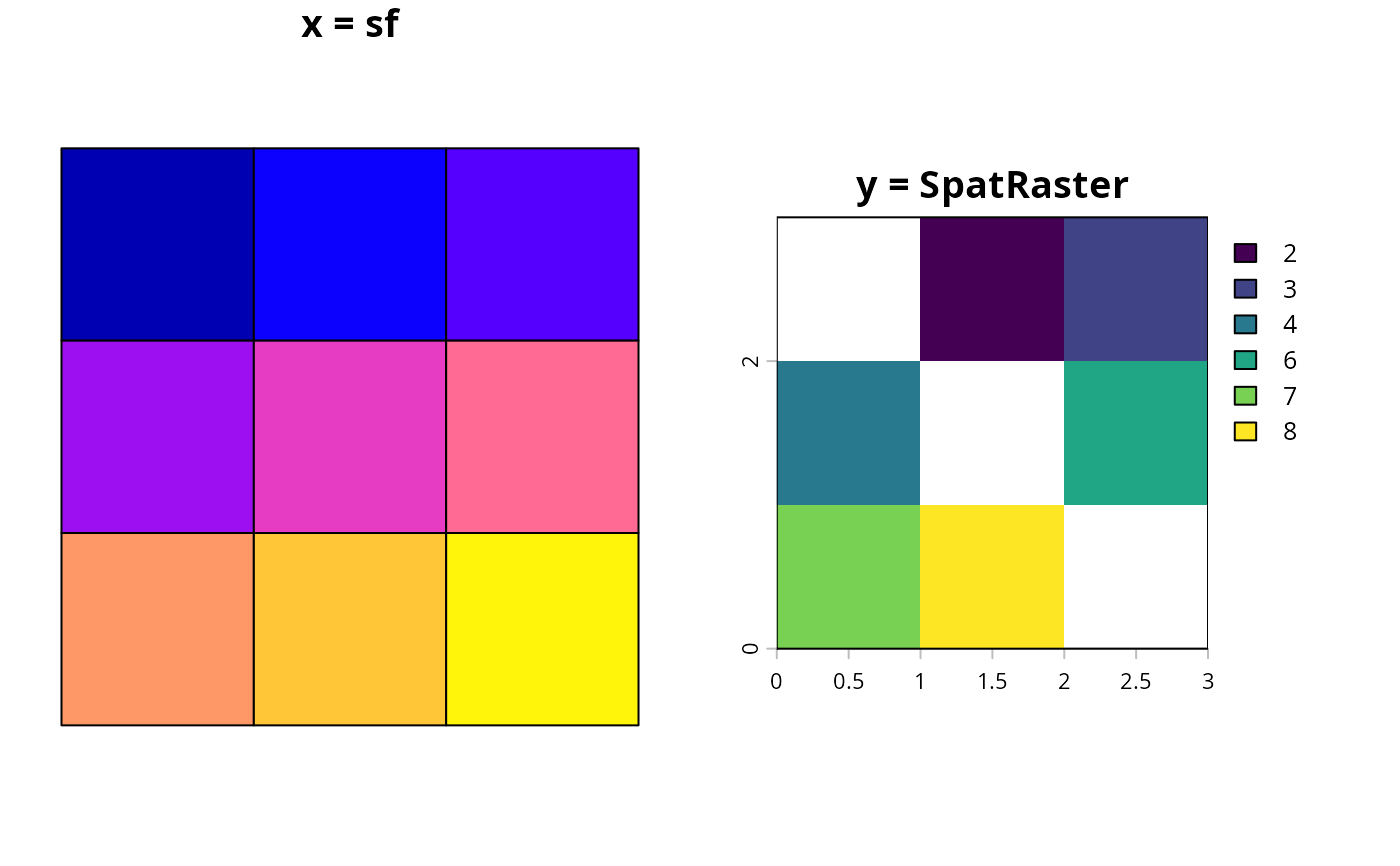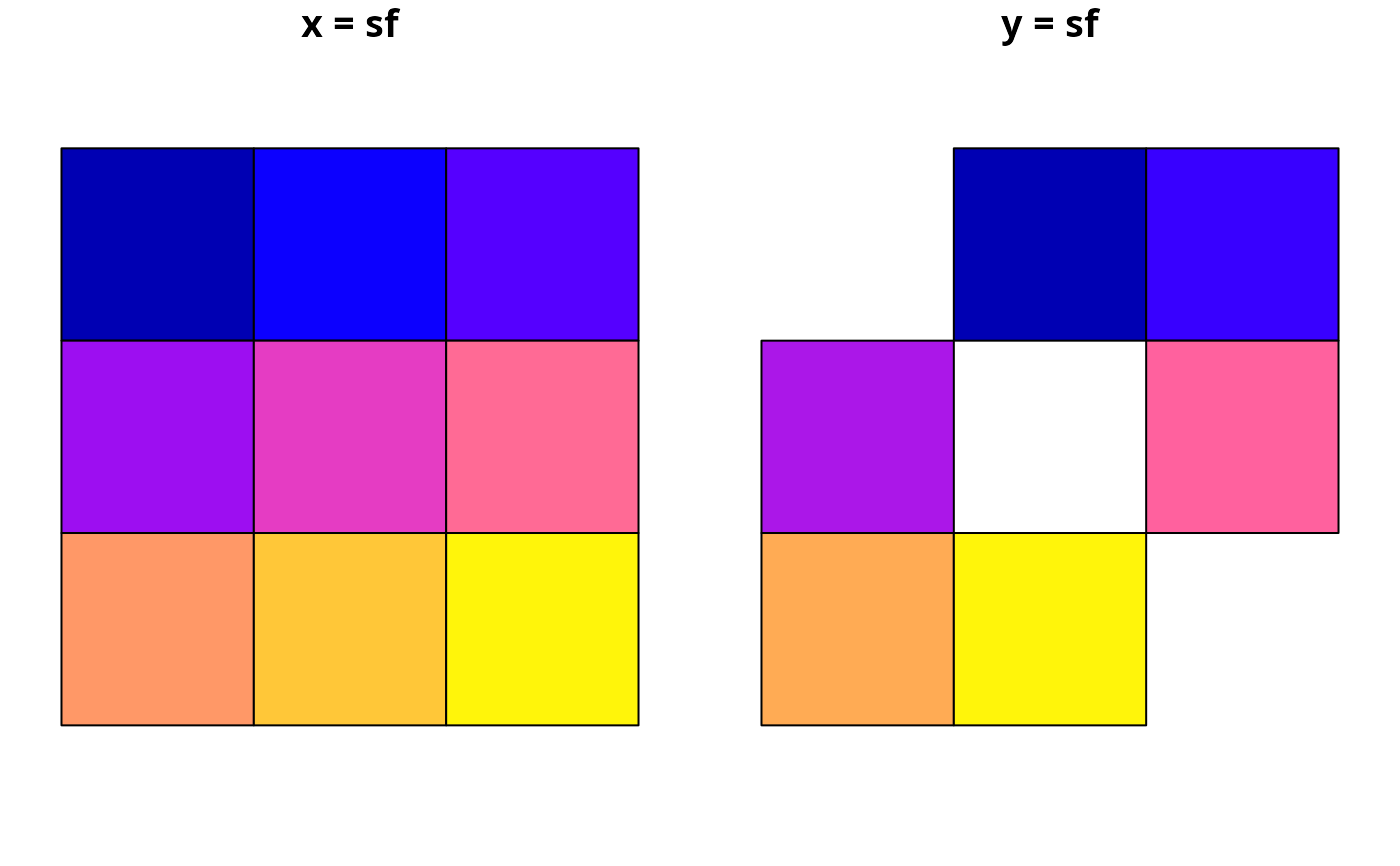Find which of the units in a spatial data object intersect with the units in another spatial data object.

intersecting_units(x, y)

# S4 method for Raster,Raster
intersecting_units(x, y)

# S4 method for Spatial,Spatial
intersecting_units(x, y)

# S4 method for sf,Spatial
intersecting_units(x, y)

# S4 method for Spatial,Raster
intersecting_units(x, y)

# S4 method for Spatial,sf
intersecting_units(x, y)

# S4 method for Raster,Spatial
intersecting_units(x, y)

# S4 method for sf,sf
intersecting_units(x, y)

# S4 method for Raster,sf
intersecting_units(x, y)

# S4 method for sf,Raster
intersecting_units(x, y)

# S4 method for data.frame,ANY
intersecting_units(x, y)

## Arguments

x

Spatial or Raster object.

y

Spatial or Raster object.

## Value

integer indices of the units in x that intersect with y.

fast_extract().

## Examples

# create data
r <- raster(matrix(1:9, byrow = TRUE, ncol=3))
r_with_holes <- r
r_with_holes[c(1, 5, 9)] <- NA
ply <- rasterToPolygons(r)
ply_with_holes <- st_as_sf(rasterToPolygons(r_with_holes))

# intersect raster with raster
# \dontrun{
par(mfrow = c(1, 2))
plot(r, main = "x=Raster")
plot(r_with_holes, main = "y=Raster")# }
print(intersecting_units(r, r_with_holes))
#>  2 3 4 6 7 8

# intersect raster with polygons (sf)
# \dontrun{
par(mfrow = c(1, 2))
plot(r, main = "x=Raster")
plot(ply_with_holes, main = "y=sf", key.pos = NULL, reset = FALSE)# }
print(intersecting_units(r, ply_with_holes))
#>  2 3 4 6 7 8

# intersect polygons (Spatial) with raster
# \dontrun{
par(mfrow = c(1, 2))
plot(ply, main = "x=Spatial")
plot(r_with_holes, main = "y=Raster")# }
print(intersecting_units(ply, r_with_holes))
#>  2 3 4 5 6 7 8

# intersect polygons (Spatial) with polygons (sf)
# \dontrun{
par(mfrow = c(1, 2))
plot(ply, main = "x=Spatial")
plot(ply_with_holes, main = "y=sf", key.pos = NULL, reset = FALSE)# }
print(intersecting_units(ply, ply_with_holes))
#>  2 3 4 6 7 8